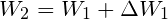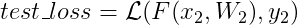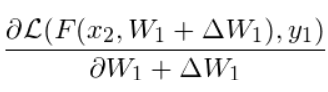# How to compute 2nd derivative of the loss computing from a fine-tuned model w.r.t pre-trained model's input?

I’m new to Pytorch. I have a pre-trained model W1 and two data point (x1, y1), (x2, y2). Firstly, I fine-tune W1 with (x1, y1) in one epoch, thus the model updatecan be obtained by doingAnd then, I get the test loss for (x2, y2) w.r.t fine-tuned modelbyWhat I want to do is to use the test_loss to update x1 by doing

Here I offer a toy code where the model is F(x)=wx, and the two data points are (1, 1) and (2, 2) respectively.

``````import torch, torch.nn as nn

torch.manual_seed(1)
torch.cuda.manual_seed(1)

class Net(nn.Module):
def __init__(self):
super(Net, self).__init__()
self.fc_blocks = nn.Sequential(
nn.Linear(1, 1, bias=False)
)
def forward(self, x):
output = self.fc_blocks(x)
return output

def weight_init(m):
if isinstance(m, nn.Linear):
nn.init.constant_(m.weight, 2.0)

net = Net()
net.apply(weight_init)
criterion = nn.MSELoss()

x_1 = torch.tensor([[1.0]], requires_grad=True)
y_1 = torch.tensor([[1.0]])
x_2 = torch.tensor([[2.0]])
y_2 = torch.tensor([[2.0]])

x_optimizer = torch.optim.SGD([x_1,], lr = 1)
net_optimizer = torch.optim.SGD(net.parameters(), lr = 1)

for i in range(1):
def closure():
y_pred = net(x_1)
pred_loss = criterion(y_pred, y_1)
dp_dw = grad(pred_loss, net.parameters(), create_graph=True, retain_graph=True)
dp_dw.backward()

for param in net.parameters():
param.data = param.data - param.grad.data

y_test = net(x_2)
test_loss = criterion(y_test, y_2)
test_loss.backward(create_graph=True)
return test_loss
x_optimizer.step(closure)
``````

And the result is

``````\$ python test.py
``````

It seems that the x1.grad doesn’t change in step2 mainly caused by the the computation graph forgetting this operation. But I have manually re-assign the model’s parameters intead of using in-place operation implemented in `step()` function.
What am I doing wrong here? Any help is appreciated. Thank you.

Hi,

The main problem you encounter here is that the `nn.Module` and `torch.optim` modules are not built to do what you want. In particular, the parameters of the modules and the optimizers are built such that weight updates are not differentiated through.

The two issues you want to fix here are:

• You don’t want to use builtin optimizers as they perform their updates in a non-differentiable way.
• You don’t want to use vanilla modules because their weights are `nn.Parameter` for which you never track history (they are always leaf Tensors).

If you only want to perform testing for research purposes, I would advise

• Create regular pytorch modules.
• Create the new base parameters as `mod.true_weight = nn.Parameter(mod.weight)`
• Delete all the old parameters by doing `del mod.weight` manually.
• Have a way to manually set the `.weight` to the base parameters (that will use only for your first iteration)
• For every param, do `mod.weight = mod.true_weight.view_as(mod.true_weight)` (do an operation to make sure you don’t get a new Tensor (that share memory)).
• Have a custom optimizers that will do in a differentiable way the weight update based on the true_weight’s gradients: `mod.weight = mod.weight + lr * mod.true_weight.grad`.
• Use the regular `mod(inp)` to do the forward calls.

That way, you will be able to manually do these different iterations.

3 Likes

Thanks for your patient reply and please forgive my slow response.

I know the idea in your operations, but I don’t know how to implement it. Could you please provide me some specific examples?

By the way, I wonder if it’s possible to manually compute the gradient

By `dp_dw.backward()` and `x_1.grad` in the first iteration, I can get the gradient(Is it correct since \Delta W1 is a function of x1 while W1 is not a function of x? ) And it’s easy to get the gradientwith `test_loss.backward()` and `net.parameters().grad` in the second iteration. So can I pass the manually computed gradient to a optimizer in order to optimize x1?